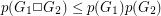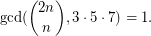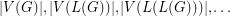# Graham, Ronald L.

## Termination of the sixth Goodstein Sequence ★

Author(s): Graham

Question   How many steps does it take the sixth Goodstein sequence to terminate?

Keywords: Goodstein Sequence

## Monotone 4-term Arithmetic Progressions ★★

Author(s): Davis; Entringer; Graham; Simmons

Question   Is it true that every permutation of positive integers must contain monotone 4-term arithmetic progressions?

## Pebbling a cartesian product ★★★

Author(s): Graham

We letdenote the pebbling number of a graph.

Conjecture.

Keywords: pebbling; zero sum

## Divisibility of central binomial coefficients ★★

Author(s): Graham

Problem  (1)   Prove that there exist infinitely many positive integerssuch thatProblem  (2)   Prove that there exists only a finite number of positive integerssuch thatKeywords:

## The large sets conjecture ★★★

Author(s): Brown; Graham; Landman

Conjecture   Ifis 2-large, thenis large.

Keywords: 2-large sets; large sets

## Graham's conjecture on tree reconstruction ★★

Author(s): Graham

Problem   for every graph, we letdenote the line graph of. Given thatis a tree, can we determine it from the integer sequence?

Keywords: reconstruction; tree